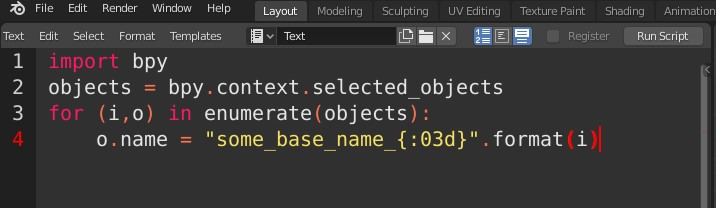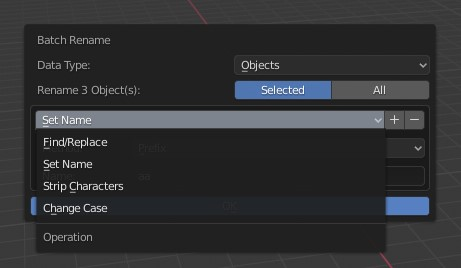## A collection of Python snippets for 3D

If you’re interested in taking the first step into Python for 3D software,
Or simply would like to browse some script examples, your welcome to visit my Gist page,
It contains a useful library of Python code example for Blender, Maya, 3ds max and Unreal engine:
https://gist.github.com/CGLion

## Blender Python – Reading mesh UV data

Software:
Blender 2.83

Simple example code for reading mesh UV data.

Link to this code snippet on gist

Note that there are typically many more mesh loops than vertices.
*Unless the case is a primitive undivided plane..

``````import bpy
access mesh data:
obj = bpy.context.active_object
mesh_data = obj.data
mesh_loops = mesh_data.loops
uv_index = 0
iterate teh mesh loops:
for lp in mesh_loops:
# access uv loop:
uv_loop = mesh_data.uv_layers[uv_index].data[lp.index]
uv_coords = uv_loop.uv
print('vert: {}, U: {}, V: {}'.format(lp.vertex_index,      uv_coords, uv_coords))``````

## Blender – Python – Access animated vertices data

Software:
Blender 2.83

The following is simple example of reading a mesh’s animated vertices data.

This example code gist

Note that accessing an model’s animated vertex locations requires reading the model’s evaluated (deformed) mesh state per frame.
For that reason a new bmesh object is initiated per each frame with the the model’s updated dependency graph.

``````import bpy
import bmesh
obj = bpy.context.active_object
frames = range(0,10)
get the object's evaluated dependency graph:
depgraph = bpy.context.evaluated_depsgraph_get()
iterate animation frames:
for f in frames:
bpy.context.scene.frame_set(f)
# define new bmesh object:
bm = bmesh.new()
bm.verts.ensure_lookup_table()
# read the evaluated mesh data into the bmesh   object:
bm.from_object( obj, depgraph )
# iterate the bmesh verts:
for i, v in enumerate(bm.verts):
print("frame: {}, vert: {}, location: {}".format(f, i, v.co))``````

## Python for Blender – Batch renaming objects

Software:
Blender 2.8 | Python 3.7

Some useful short Python snippets for batch re-naming objects in Blender:

1. Remove last 4 characters from selected object names:
```import bpy
objects = bpy.context.selected_objects
for o in objects:
o.name = o.name[:-4]```2. Rename all selected objects to a set base name followed by a 3 digit numeric suffix:
```import bpy
objects = bpy.context.selected_objects
for (i,o) in enumerate(objects):
o.name = "some_base_name_{:03d}".format(i)```3. Prefix all selected objects name with their Blender data type like renaming “SomeModel” to “MESH_SomeModel” or for example:
```import bpy
objects = bpy.context.selected_objects
for (i,o) in enumerate(objects):
o.name = "{}_{}".format(o.type,o.name)```* note that when copying and pasting a script from this example, the indentation may not be pasted correctly.

Note:
Blender 2.8 has a robust batch renaming utility that is invoked by pressing Ctrl + F2 so we don’t have to write scripts to do batch renaming of objects:## Blender Python – Accessing mesh triangles

Software:
Blender 2.81 | Python 3.74

By default, mesh triangles are not accessible to Python in Blender.
When accessing the mesh triangles is needed, they must be calculated first using the ‘calc_loop_triangles’ Mesh object method.
Before the calc_loop_triangle method has been called, the loop_triangles property of the Mesh object will reference a empty collection.
After calling the calc_loop_triangles method, the loop_triangles property will reference a collection of MeshLoopTriangle objects, in which the vertices property will hold an array of 3 integers that are the indices of the triangle vertices.

The following example script creates and places sphere objects at the centers of the cube’s triangles:

```import bpy
mesh = bpy.data.objects['Cube'].data
mesh.calc_loop_triangles()
for tri in mesh.loop_triangles:
tri_center = (mesh.vertices[tri.vertices].co * 0.333) +\
(mesh.vertices[tri.vertices].co * 0.333) +\
(mesh.vertices[tri.vertices].co * 0.333)
enter_editmode=False,
location=tri_center)```

* note that when copying and pasting a script from this example, the indentation may not be pasted correctly.

## Blender Python – Make an unselectable object local so it can be selected..

Software:
Blender 2.79

If you happen to link an external object that was made unselectable in the original blend file, You will have no interactive way to make it selectable again, or local, or even delete it from the current blend file.

The good news is that it can easily be done by a very short Python script:

```import bpy

obj = bpy.data.objects['the_untouchable_object']
obj.make_local()```

It should also be very easy script a loop that will find all those linked objects,
I will post an example when I’ll have a minute to breathe… 😀